Transcripted Summary

So far, most of the methods that we've looked at throughout the course have taken a primitive data type as its parameters. And when we say primitive data type this means things like the `int` and the `char`.

However, we can use objects as the arguments for methods, as well.

Let's show some examples. We're going to redo this `HomeAreaCalculator` class.

I made a new one that's called `HomeAreaCalculatorRedo`:

``````package chapter6;

public class HomeAreaCalculatorRedo {

public static void main(String args[]){

}
}
``````

And we're going to create two instances of the rectangle again as rooms, but we'll make this a little bit more understandable.

We'll call this 1st one `kitchen`, and we'll make a call to a method we haven't defined yet called `getRoom()`.

``````public static void main(String args[]){

Rectangle kitchen = getRoom();
}
``````

And let's make one more rectangle; we'll call this `bathroom`:

``````public static void main(String args[]){

Rectangle kitchen = getRoom();
Rectangle bathroom = getRoom();
}
``````

Let's go ahead and define this `getRoom()` method.

This method is not going to return a primitive data type. It's going to return the `Rectangle` object, because that's what we're expecting from `getRoom()`. We see that we don't have to limit our methods to the data types that it returns being primitive, nor the parameters that it accepts.

``````public Rectangle getRoom(){

}
``````

Now inside of this method, let's prompt the user to enter the length and the width of their room. First we need to create the `scanner`.

Let's highlight this `Scanner` class.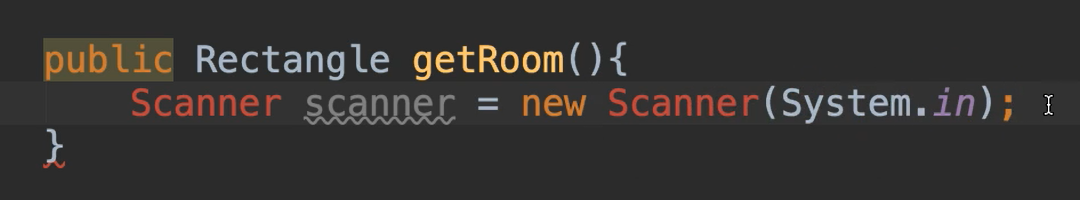We've been using this all along and now it should look really familiar to you what we were doing. So, the `Scanner` is a class that's already defined in Java. We are using `scanner` [the lower case one] as our object name. We're using `new` to instantiate this. And we're calling a constructor that takes in this argument.

Now, we're going to go ahead and import this.

``````import java.util.Scanner;

public class HomeAreaCalculatorRedo {
``````

Now notice we had to import the `Scanner` class, but we didn't have to import the `Rectangle` class.

Why is that? It's because `Rectangle` is in the same package as this current class, `HomeAreaCalculatorRedo`. However, `Scanner` belongs to `java.util` package. Because it's in a different package, that's why we have to import it.

Why do we have to import some classes?

For classes that are not within the same package, you have to import them.

Let me go ahead and type out the prompts to get the length and width now.

``````public Rectangle getRoom(){
Scanner scanner = new Scanner(System.in);

System.out.println("Enter the length of your room:");
double length = scanner.nextDouble();

System.out.println("Enter the width of your room:");
double width = scanner.nextDouble();

scanner.close();
}
``````

Now remember in the last chapter when we talked about methods, I told you that a method can only return one value. And we even had a case where we had 2 values that we wanted returned, but we had to split that method up.

Now that we're using objects, we no longer have to do that sort of thing. So, we can have this `length` and have this `width`, and it's part of an overall object. So, we're going to return one value back, but that value happens to be an object that contains lots of different data. So that's a really good perk.

So, we're going to create this new `Rectangle`.

And actually, I'm going to show you something new. I can create this `Rectangle` object how we've done in the previous section, or I can just return the new instantiation as opposed to storing it in a new object. don't need to store this.

Here’s what it would look like if I created the object then returned it.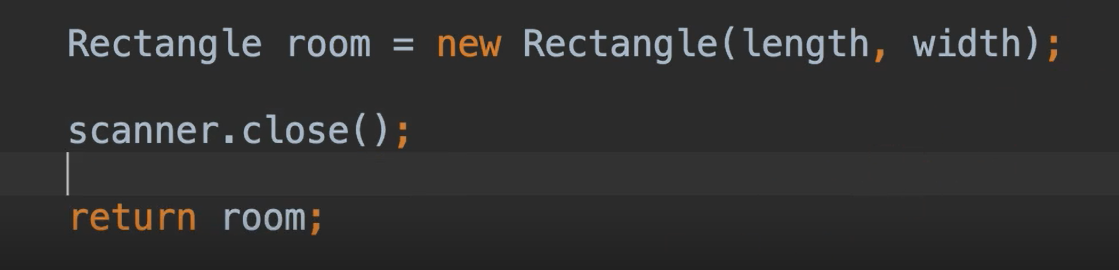This is perfectly fine; however, we are declaring this new object and we never use it again except for to return. So, it's kind of a waste to store this.

Instead we can do a little shortcut and just say this:

``````return new Rectangle(length, width);
``````

So, we don't have to assign it. We can instantiate this and then return it like this. That's legal as well.

Now we have our `getRoom()` method. And we're making calls to this `getRoom()` method. However, it's complaining there's a compilation error.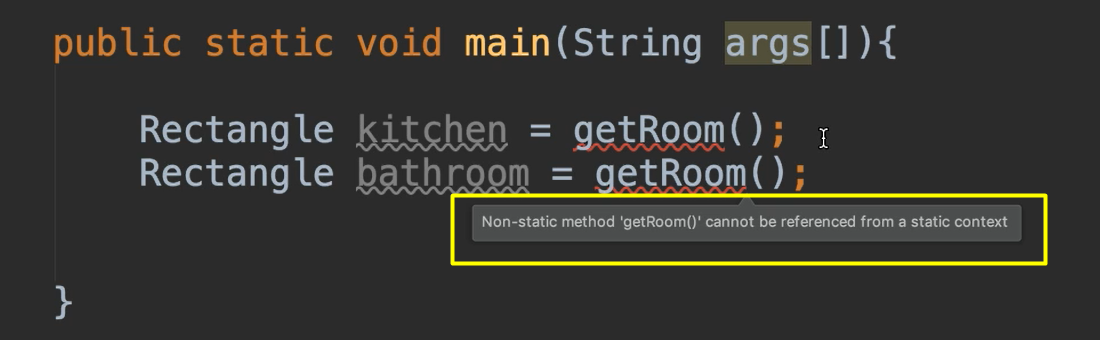If we look at this compilation error, it's saying that the non-static method `getRoom()` cannot be referenced from a static context.

So far within our classes, we've been using this `static void main`. Now `main` has to be `static` — that's the way Java is built.

However, all of our other things don't necessarily have to be `static`. And now that we've talked about classes and objects, we can talk a little bit more about `static`.

Static Non-Access Modifier

`Static` is used for members of a class which can be accessed without using an instance of the class to do so. Because it’s not associated with an instantiation, these members have no state.

Let's look at our class from a little while ago, `HomeAreaCalculator`: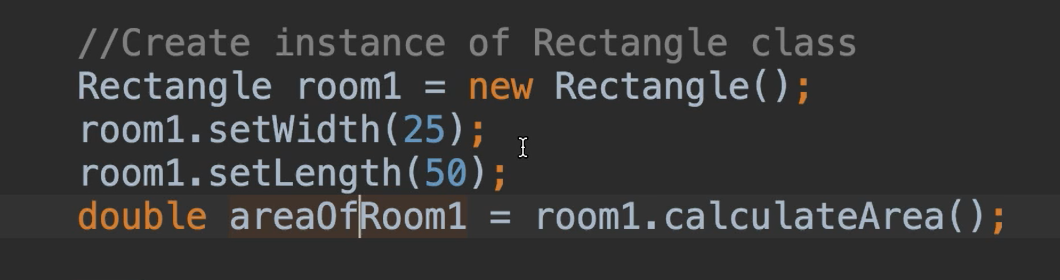When we set this `width` and we set this `length`, we've now set state for `room1`. When we want to call `calculateArea()`, we didn't have to pass in any additional information because we've already set this up as state. So, we can make subsequent calls and it's keeping track of all the data that we've already set for this object.

In a `static` context, there's no such thing as state — you're just calling to a method itself.

If we don't want to use `static` and we want to keep state, then what we would need to do is create an instance and then call the methods.

Now we're within this same class, but in order to call `getRoom()` without making `getRoom()` static, we would have to instantiate the very class that we are in. So, let's do that above here [where we called `getRoom()`] and we’ll call this “calculator”:

``````HomeAreaCalculatorRedo calculator = new HomeAreaCalculatorRedo();
Rectangle kitchen = getRoom();
Rectangle bathroom = Room();
``````

Now that we have that, we can use this `calculator` object and use the dot operator to call the `getRoom()` method:

``````HomeAreaCalculatorRedo calculator = new HomeAreaCalculatorRedo();
Rectangle kitchen = calculator.getRoom();
Rectangle bathroom = calculator.getRoom();
``````

So, we've created an instance of the very class that we were in, just so that we could get the things that are not `static` within this class.

Notice something else that we've done here. We've instantiated this new `HomeAreaCalculatorRedo` by calling this default constructor. But there's no such constructor defined in this class.

That's because in Java a default constructor is always defined implicitly.

So, even if you do not declare it explicitly, it still exists by default under the covers. Essentially it’s a blank constructor that does nothing.

Okay, now we have our kitchen and we have our bathroom, so let's go ahead and calculate the area.

We'll say `double area =` and we'll make a call to yet another method that we're going to define. And this method is going to take two rectangles:

``````double area = calculateTotalArea(kitchen, bathroom);
``````

Let's go ahead and define that method now — it needs to return a `double`, and it needs to accept rectangles.

``````public double calculateTotalArea(Rectangle rectangle1, Rectangle rectangle2){

}
``````

So inside of here, now we have two objects that we've accepted. We've declared these as our parameter list.

And now we can use the dot operator how we see fit.

So, we want to calculate the area — the area is the area of this rectangle plus the area of this rectangle.

``````public double calculateTotalArea(Rectangle rectangle1, Rectangle rectangle2){
return rectangle1.calculateArea() + rectangle2.calculateArea();
}
``````

And this is complaining again, because we did not use our `calculator` object to access this non-static method.

Let’s correct this:

``````double area = calculator.calculateTotalArea(kitchen, bathroom);
``````

Okay, so now we've done the area.

Let’s print it out.

``````System.out.println("The total area is: " + area);
``````

Okay, we'll give this a go.

And we'll say 30, 25.

Ooh, we got an exception here.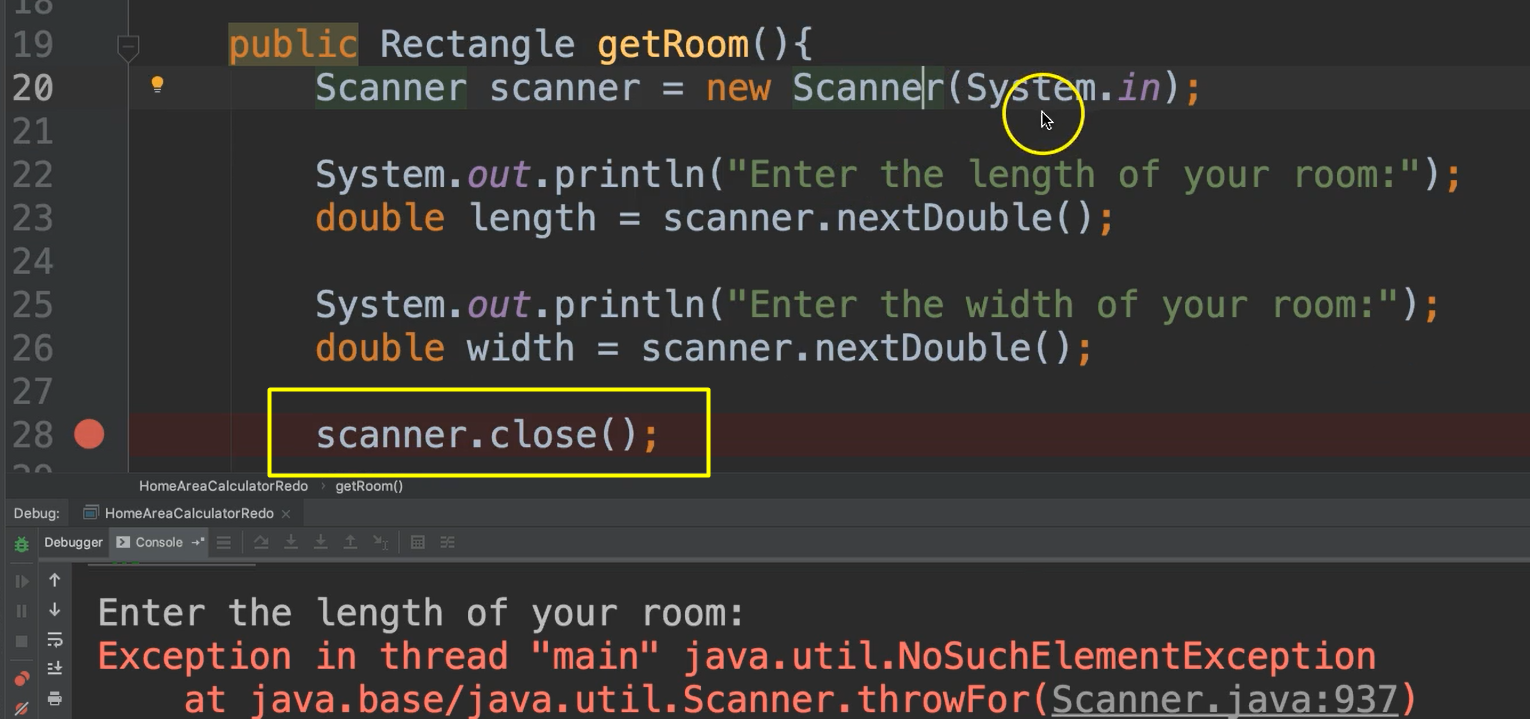This is because we need to use this `scanner` object twice, but we close it after the first use. The first time we called `getRoom()`, we instantiated `scanner`, got the input, and then it closed it. But when it closes this, it’s doing something advanced with this `System.in`.

In order to fix this, we need to just put this [`scanner.close();`] in our `main` method so that we only close the `scanner` one time throughout our entire program. So, the declaration of `Scanner` needs to be global if we're going to use it in multiple places, so let's do that.

And then let's move this [`scanner.close();] to the very end of the`main` method.

And `scanner` is not `static`, so it cannot be used directly in static `main`. So, what do we do?

We have to use `calculator` to access it:

``````calculator.scanner.close();
``````

So, this is not only used for the methods, it can also be used for the fields, which in this case is also an object.

Let's also make our `scanner` private:

``````private Scanner scanner = new Scanner(System.in);
``````

### # HomeAreaCalculatorRedo.java

``````package chapter6;

import java.util.Scanner;

public class HomeAreaCalculatorRedo {

private Scanner scanner = new Scanner(System.in);

public static void main(String args[]){

HomeAreaCalculatorRedo calculator = new HomeAreaCalculatorRedo();
Rectangle kitchen = calculator.getRoom();
Rectangle bathroom = calculator.getRoom();

double area = calculator.calculateTotalArea(kitchen, bathroom);

System.out.println("The total area is: " + area);

calculator.scanner.close();
}

public Rectangle getRoom(){

System.out.println("Enter the length of your room:");
double length = scanner.nextDouble();

System.out.println("Enter the width of your room:");
double width = scanner.nextDouble();

return new Rectangle(length, width);
}

public double calculateTotalArea(Rectangle rectangle1, Rectangle rectangle2){
return rectangle1.calculateArea() + rectangle2.calculateArea();
}
}
``````

And let's run it — 30 and 25, and let's say, 50 and 75. And we get 4500, okay. So good.

In this section we learned about `static` and we learned about using an object as return type and also as parameters to a method.

Great job.